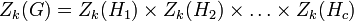# Upper central series may be tight with respect to nilpotency class

(diff) ← Older revision | Latest revision (diff) | Newer revision → (diff)

## Statement

Let$c$ be any natural number. Then, we can construct a nilpotent group$G$ of nilpotency class$c$ with the following property.

Let$Z_k(G)$ denote the$k^{th}$ member of the Upper central series (?) of$G$:$Z_1(G) = Z(G)$ is the center and$Z_k(G)/Z_{k-1}(G)$ is the center of$G/Z_{k-1}(G)$ for all$k$. By definition of Nilpotency class (?),$Z_c(G) = G$.

We can find a$G$ with the property that for any$k \le c$,$Z_k(G)$ has nilpotency class precisely$k$.

## Related facts

### Opposite facts for lower central series

The corresponding statement is not true for the lower central series. Some related facts:

### Opposite facts for upper central series

It is also true that the upper central series for any member of the upper central series (beyond the center) grows faster than the actual upper central series of the whole group. See:

## Proof

Let$H_1, H_2, \dots H_c$ be groups such that each$H_k$ is a nilpotent group of nilpotency class precisely$k$, i.e., it is not nilpotent of class smaller than$k$. Define$G$ as the external direct product:$G = H_1 \times H_2 \times \dots \times H_c$

Now, for each$k$, we have:$Z_k(G) = Z_k(H_1) \times Z_k(H_2) \times \dots \times Z_k(H_c)$

In particular, we obtain that:$Z_k(G) = H_1 \times H_2 \times \dots \times H_k \times Z_k(H_{k+1}) \times \dots \times Z_k(H_c)$

From the given data, in particular the fact that$H_k$ has nilpotency class exactly$k$, it is clear that$Z_k(G)$ has nilpotency class exactly$k$.